# sklearn.impute.SimpleImputer¶

class sklearn.impute.SimpleImputer(*, missing_values=nan, strategy='mean', fill_value=None, verbose='deprecated', copy=True, add_indicator=False, keep_empty_features=False)[source]

Univariate imputer for completing missing values with simple strategies.

Replace missing values using a descriptive statistic (e.g. mean, median, or most frequent) along each column, or using a constant value.

Read more in the User Guide.

New in version 0.20: SimpleImputer replaces the previous sklearn.preprocessing.Imputer estimator which is now removed.

Parameters:
missing_valuesint, float, str, np.nan, None or pandas.NA, default=np.nan

The placeholder for the missing values. All occurrences of missing_values will be imputed. For pandas’ dataframes with nullable integer dtypes with missing values, missing_values can be set to either np.nan or pd.NA.

strategystr, default=’mean’

The imputation strategy.

• If “mean”, then replace missing values using the mean along each column. Can only be used with numeric data.

• If “median”, then replace missing values using the median along each column. Can only be used with numeric data.

• If “most_frequent”, then replace missing using the most frequent value along each column. Can be used with strings or numeric data. If there is more than one such value, only the smallest is returned.

• If “constant”, then replace missing values with fill_value. Can be used with strings or numeric data.

New in version 0.20: strategy=”constant” for fixed value imputation.

fill_valuestr or numerical value, default=None

When strategy == “constant”, fill_value is used to replace all occurrences of missing_values. For string or object data types, fill_value must be a string. If None, fill_value will be 0 when imputing numerical data and “missing_value” for strings or object data types.

verboseint, default=0

Controls the verbosity of the imputer.

Deprecated since version 1.1: The ‘verbose’ parameter was deprecated in version 1.1 and will be removed in 1.3. A warning will always be raised upon the removal of empty columns in the future version.

copybool, default=True

If True, a copy of X will be created. If False, imputation will be done in-place whenever possible. Note that, in the following cases, a new copy will always be made, even if copy=False:

• If X is not an array of floating values;

• If X is encoded as a CSR matrix;

• If add_indicator=True.

If True, a MissingIndicator transform will stack onto output of the imputer’s transform. This allows a predictive estimator to account for missingness despite imputation. If a feature has no missing values at fit/train time, the feature won’t appear on the missing indicator even if there are missing values at transform/test time.

keep_empty_featuresbool, default=False

If True, features that consist exclusively of missing values when fit is called are returned in results when transform is called. The imputed value is always 0 except when strategy="constant" in which case fill_value will be used instead.

New in version 1.2.

Attributes:
statistics_array of shape (n_features,)

The imputation fill value for each feature. Computing statistics can result in np.nan values. During transform, features corresponding to np.nan statistics will be discarded.

indicator_MissingIndicator

Indicator used to add binary indicators for missing values. None if add_indicator=False.

n_features_in_int

Number of features seen during fit.

New in version 0.24.

feature_names_in_ndarray of shape (n_features_in_,)

Names of features seen during fit. Defined only when X has feature names that are all strings.

New in version 1.0.

IterativeImputer

Multivariate imputer that estimates values to impute for each feature with missing values from all the others.

KNNImputer

Multivariate imputer that estimates missing features using nearest samples.

Notes

Columns which only contained missing values at fit are discarded upon transform if strategy is not "constant".

In a prediction context, simple imputation usually performs poorly when associated with a weak learner. However, with a powerful learner, it can lead to as good or better performance than complex imputation such as IterativeImputer or KNNImputer.

Examples

>>> import numpy as np
>>> from sklearn.impute import SimpleImputer
>>> imp_mean = SimpleImputer(missing_values=np.nan, strategy='mean')
>>> imp_mean.fit([[7, 2, 3], [4, np.nan, 6], [10, 5, 9]])
SimpleImputer()
>>> X = [[np.nan, 2, 3], [4, np.nan, 6], [10, np.nan, 9]]
>>> print(imp_mean.transform(X))
[[ 7.   2.   3. ]
[ 4.   3.5  6. ]
[10.   3.5  9. ]]


Methods

 fit(X[, y]) Fit the imputer on X. fit_transform(X[, y]) Fit to data, then transform it. get_feature_names_out([input_features]) Get output feature names for transformation. get_params([deep]) Get parameters for this estimator. Convert the data back to the original representation. set_output(*[, transform]) Set output container. set_params(**params) Set the parameters of this estimator. Impute all missing values in X.
fit(X, y=None)[source]

Fit the imputer on X.

Parameters:
X{array-like, sparse matrix}, shape (n_samples, n_features)

Input data, where n_samples is the number of samples and n_features is the number of features.

yIgnored

Not used, present here for API consistency by convention.

Returns:
selfobject

Fitted estimator.

fit_transform(X, y=None, **fit_params)[source]

Fit to data, then transform it.

Fits transformer to X and y with optional parameters fit_params and returns a transformed version of X.

Parameters:
Xarray-like of shape (n_samples, n_features)

Input samples.

yarray-like of shape (n_samples,) or (n_samples, n_outputs), default=None

Target values (None for unsupervised transformations).

**fit_paramsdict

Returns:
X_newndarray array of shape (n_samples, n_features_new)

Transformed array.

get_feature_names_out(input_features=None)[source]

Get output feature names for transformation.

Parameters:
input_featuresarray-like of str or None, default=None

Input features.

• If input_features is None, then feature_names_in_ is used as feature names in. If feature_names_in_ is not defined, then the following input feature names are generated: ["x0", "x1", ..., "x(n_features_in_ - 1)"].

• If input_features is an array-like, then input_features must match feature_names_in_ if feature_names_in_ is defined.

Returns:
feature_names_outndarray of str objects

Transformed feature names.

get_params(deep=True)[source]

Get parameters for this estimator.

Parameters:
deepbool, default=True

If True, will return the parameters for this estimator and contained subobjects that are estimators.

Returns:
paramsdict

Parameter names mapped to their values.

inverse_transform(X)[source]

Convert the data back to the original representation.

Inverts the transform operation performed on an array. This operation can only be performed after SimpleImputer is instantiated with add_indicator=True.

Note that inverse_transform can only invert the transform in features that have binary indicators for missing values. If a feature has no missing values at fit time, the feature won’t have a binary indicator, and the imputation done at transform time won’t be inverted.

New in version 0.24.

Parameters:
Xarray-like of shape (n_samples, n_features + n_features_missing_indicator)

The imputed data to be reverted to original data. It has to be an augmented array of imputed data and the missing indicator mask.

Returns:
X_originalndarray of shape (n_samples, n_features)

The original X with missing values as it was prior to imputation.

set_output(*, transform=None)[source]

Set output container.

See Introducing the set_output API for an example on how to use the API.

Parameters:
transform{“default”, “pandas”}, default=None

Configure output of transform and fit_transform.

• "default": Default output format of a transformer

• "pandas": DataFrame output

• None: Transform configuration is unchanged

Returns:
selfestimator instance

Estimator instance.

set_params(**params)[source]

Set the parameters of this estimator.

The method works on simple estimators as well as on nested objects (such as Pipeline). The latter have parameters of the form <component>__<parameter> so that it’s possible to update each component of a nested object.

Parameters:
**paramsdict

Estimator parameters.

Returns:
selfestimator instance

Estimator instance.

transform(X)[source]

Impute all missing values in X.

Parameters:
X{array-like, sparse matrix}, shape (n_samples, n_features)

The input data to complete.

Returns:
X_imputed{ndarray, sparse matrix} of shape (n_samples, n_features_out)

X with imputed values.

## Examples using sklearn.impute.SimpleImputer¶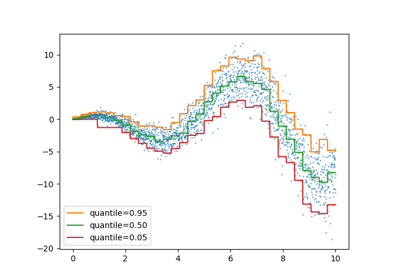Release Highlights for scikit-learn 1.1

Release Highlights for scikit-learn 1.1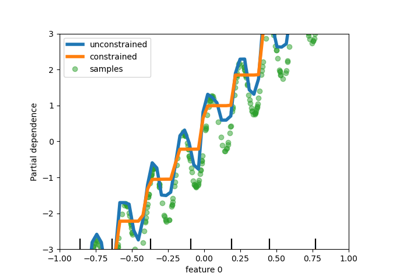Release Highlights for scikit-learn 0.23

Release Highlights for scikit-learn 0.23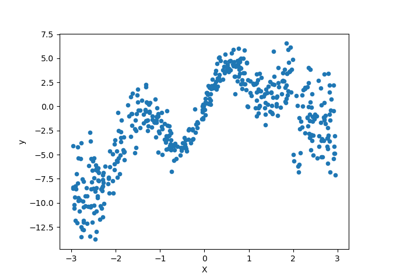Combine predictors using stacking

Combine predictors using stacking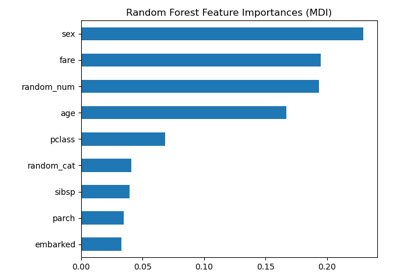Permutation Importance vs Random Forest Feature Importance (MDI)

Permutation Importance vs Random Forest Feature Importance (MDI)Displaying Pipelines

Displaying PipelinesDisplaying estimators and complex pipelines

Displaying estimators and complex pipelines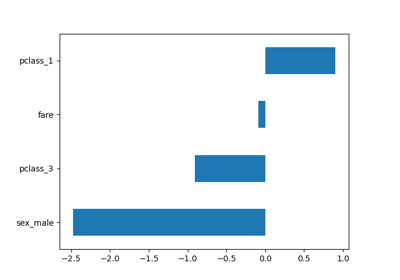Introducing the set_output API

Introducing the set_output API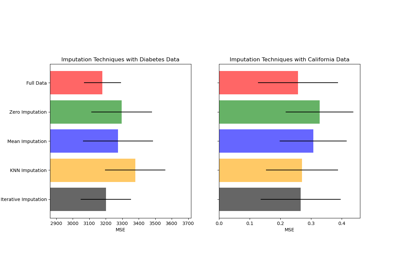Imputing missing values before building an estimator

Imputing missing values before building an estimator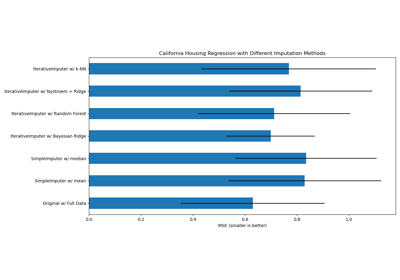Imputing missing values with variants of IterativeImputer

Imputing missing values with variants of IterativeImputerColumn Transformer with Mixed Types

Column Transformer with Mixed Types